Home Articles Improving classification accuracy using knowledge based approach

# Improving classification accuracy using knowledge based approach

Alesheikh, Ali A.
Assistant Professor
Email: [email protected]

Dept. of Geodesy and Geomatics Eng.,K.N. Toosi University of Technology
Email: [email protected]
Tel: (+98 21) 8779473,Fax: (+98 21) 8779476

Applications & GIS Director, Iranian Remote Sensing Centre

Introduction
Remotely sensed images are major sources of data and information that are used in various fields such as environmental studies, forest management, and urban change detection. One of the products of the images is a thematic map. So far many efforts have been performed to extract information from remotely sensed images and various methods have been developed in this field. One of the main approaches is quantitative analysis (digital interpretation). Among digital techniques, classification is a common and powerful information extraction method, which is used in remote sensing. There are many classification methods that have their own advantages and drawbacks. Between classification methods, maximum likelihood approach has been used more frequently. Standard classification methods usually concern pixels as main elements and try to label the pixels individually. But, their results are not perfect and always are erroneous, since many steps are introducing errors in the classification process. Initial data (pixels) have influenced by some errors. The error sources will be explained to understand better benefits of this paper.

Future work may well produce an integrating method from which a user can select a mix appropriate to the spatial, spectral, and temporal resolution of the data in hand and information output desired (Richards, 1993). The purpose of this paper is to show how some knowledge such as prior information about the expected distribution of classes in a final classification map can be used to improve classification accuracies. Prior information is incorporated through the use of prior probabilities-that is, probabilities of occurrence of classes which are based on separate, independent knowledge concerning the area to be classified. Used in their simplest form, the probabilities weight the classes according to their expected distribution in the output dataset by shifting decision space boundaries to produce larger volumes in measurement space for classes that are expected to be large and smaller volumes for classes expected to be small.

Error Sources
Initial data (pixels) have influenced by some errors. The error sources will be:

a) During data acquisition process:
Data acquisition process in remote sensing affects the reflectance measured by the sensor and then some errors are introduced in the entered data, ands subsequently into the classification procedure. Atmosphere as a medium for transforming the energy from sun to the objects and from objects to the sensors, can affect the recorded brightness values. Atmospheric layers change the pixel brightness values in two ways, absorption and scattering. These two effects, change real brightness values, and therefore disturb the classification accuracy. But this error can be neglected relative to the others. Indeed if the application is not a specific case then the atmosphere errors can be ignored and the corresponding cost of this will be negligible.

Sensors as the measuring devices in data acquisition process have the major role; therefore they have direct effects on the captured data. Like the other devices, sensors are not perfect and thus their outputs are not as real measurements and then the brightness values are erroneous. Sensors specifications and functionality define the ultimate raw brightness values and, sensing geometry (consist of sensor situation and position) defines how three-dimension scene is transformed into two-dimension image. Spatial, spectral and radiometric resolution and particularly the Point-Spread-Function (PSF) of the sensor are the most important characteristics of the sensor that must be taken into account for classification of remotely sensed images.

b) Nature of data:
From data affect point of view, we can emphasize on three important properties of gathered data.

• Different surface materials may be distinguished by very subtle differences in their spectral patterns.
• Adjacent pixels have influence on each other and affect the brightness value of the pixels.

Land cover types do not fit into multiples of rectangular spatial units. This occurs because some objects are not similar to the pixel size. Then, the pixel may be consisting of various land cover types. This case also is in the boundaries. In this case the brightness values do not imply any certain cover type and conceptually what is called Mixels (Mixed pixels) are made.

c) During classification process:
To produce the interested results, classifiers use these data with their characteristics. Many of classification algorithms ignore the inherent data errors and assume that the input data are perfect. Standard classifiers perform the classification using only spectral properties of the image data and have not been designed for incorporating the other types of data into their process. It is clear that using the image data (spectral properties of the scene) solely, with ignoring the errors which there are in the captured data, leads to unreliable and imperfect results.

Study Area and Data
The study area is located in the North-Western of Iran, which is called Moghan (Figure 1). Moghan Agro Industry and Livestock Co has undertaken various activities in the field of crop production, horticulture, animal husbandry and related industries. About 300,000 tons of various crops such as wheat, barely, maize (seed, grain and forage), sugar beet and alfalfa is produced annually in 18000 ha of irrigated farms (Figure 2). In this research, three parts of irrigated farms are used. These three parts are flat with 2% slope.Figure 1. DEM of Moghan from 10 meters contour.

Field boundaries and their crop types are available from 1997 till 2001 (Figure 2).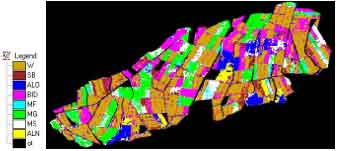Figure 2. Field boundaries of Moghan agricultural Area

The ETM+ image of the study area which was acquired on 2001-05-23 and the 1/50,000 map of it have been used in this study.

Theory and concepts:

Review of Maximum Likelihood Classification
To understand the application of prior probabilities to a classification problem, the mathematics of the maximum likelihood decision rule must be understood. For the multivariate case, we assume each observation X(pixel) consists of a set of measurements on p variables (channels). Through some external procedures, we identify a set of observations which correspond to a class-that is, a set of similar objects characterized by a vector of means on measurement variables and a variance covariance matrix describing the interrelationships among the measurement variables which are characteristics of the class (Abkar, 1999). Multivariate normal statistical theory describes the probability that an observation X will occur, given that it belongs to a class k, as the following function: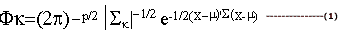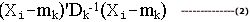can be thought as a squared distance function which measures the distance between the observation and the class mean as scaled and corrected for variance and covariance of the class. As applied in a maximum likelihood decision rule, Equation (1) allows the calculation of the probability that an observation is a member of each of k classes. The individual is then assigned to the class for which the probability value is greatest. In an operational context, observed means, variances, and covariances substituted by the log form of the Equation (1).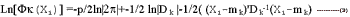Since the log of the probability is a monotonic increasing function of the probability, the decision can be made by comparing values for each class as calculated from the right hand side of this equation. A simpler decision rule, R1, can be derived from Equation (3) by eliminating the constants R1: Select k, which minimizes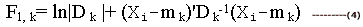The use of prior probabilities in the decision rule
The maximum likelihood decision rule can be modified easily to take into account in the population of observations as a whole. The prior probability itself is simply an estimate of the objects which will fall into a particular class. These prior probabilities are sometimes termed “weights” since the modified classification rule will tend to weigh more heavily those classes with higher prior probabilities. Prior probabilities are incorporated into the classification through manipulation of the law of Conditional Probability (Alesheikh, 1998). To begin, two probabilities are defined: P(wk), the probability that an observation will be drawn from class wk; and P(Xi), the probability of occurrence of the measurement vector Xi. The law of Conditional Probability states that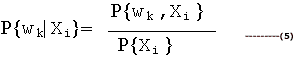the probability on the left-hand side of this expression will form the basis of a modified decision rule, since the ith observation is assigned to that class wk which has the highest probability of occurrence given the p-dimensional vector Xi which has been observed. Using the law of Conditional Probability, we find that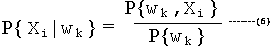In this Equation, the left-hand term describes the probability that the measurement vector will take on the values Xi given that the object measured is a member of class wk.

This probability could be determined by sampling a population of measurement vectors for observations known to be from class wk. However, the distribution of such vectors is usually assumed to be Gaussian. Thus, we can assume that P{Xiwk } is acceptably estimated by Fk(Xi)and rewrite Equation (6) as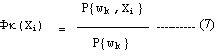Rearranging the Equation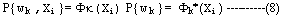Thus, the numerator of Equation (5) can be evaluated as the product of the multivariate density function Fk(Xi) and the prior probability of occurrence of class wk. To evaluate the denominator of expression (5), and knowing that for all k classes the conditional probabilities must sum to 1,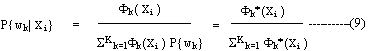This Equation provides the basis for the decision rule which includes prior probabilities. Since the denominations remain constant for all classes, the observation is simply assigned to the class for which Fk*(Xi) the product of Fk(Xi ) and P{wk}, is a maximum. In its simplest form, this decision rule can be stated as: R2: Choose k which minimizes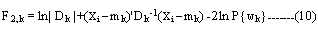It is important to understand how this decision rule behaves with different prior probabilities. If the prior probability P{wk}is very small, then its natural logarithm will be a large negative number; when multiplied by -2, it will become a large positive number and thus F 2, k for such a class will never be minimal. Therefore, setting a very small prior probability will effectively remove a class from the output classification. Note that this effect will occur even if the observation vector Xi is coincident with class mean vector mk. In such a case, the quadratic product distance function (Xi-mk)’D k-1(Xi-m k) goes to zero, but the prior probability term -2lnP{wk} can still be large. Thus, it is entirely possible that the observation will be classified into a different class, one for which the distance function is quite large.

As the prior probability P{wk} becomes large and approaches 1, its logarithm will go to zero and F2,k will approach F1,k for that class. Since this probability and all others must sum to one, however, the prior probabilities of the remaining classes will be small numbers and their values of F2,k will be greatly augmented. The effect will be to force classification into the class with high probability. Therefore, the more extreme are the values of the prior probabilities, the less important are the actual observation vector Xi.

Experimental Work
Training data for each class have been collected, and then the image is classified by maximum likelihood approach. It is assumed that a prior probability of the whole classes are equal. Figure 3 is the classified image.Figure 3. Classified image by maximum likelihood approach and equal a prior probability.

Overall accuracy of this approach is 52%. In this stage rule maps of the 8 crops can be calculated which is the basis for decision making for the software. For example Table 1 can show the rule matrix (the probability of each pixel for class W).

Table 1. Rule matrix for class W.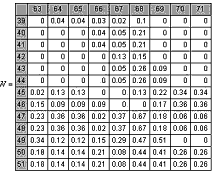Since the sum of rule matrices for whole classes must be one, Table 1 will be modified to Table .2:

Table 2. Sum of rule matrices for 8 classes.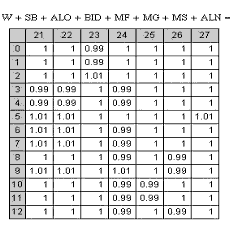Prior Probabilities Contingent on a Single External Conditioning Variable
Having shown how to modify the decision rule to take into account a set of prior probabilities, it is only a small step to consider several sets of probabilities, in which an external information source identifies which set is to be used in the decision rule. Hence, a third variable vj, is introduced, which indicates the state of the external conditioning variable (e.g. crop calendar) associated with the observation. It is expected to find an expression describing the probability that an observation will be a member of the class wk, given its vector of observed measurements and the fact that it belongs to class vj of the external conditioning variable, namely.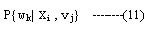In deriving an expression to find this probability, we can make the assumption that the mean vector and dispersion matrix of the class will be the same regardless of the state of the external conditioning variable. Considering this assumption and expanding Equation 11, it results in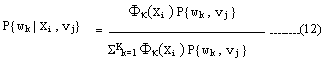This result is analogous to Equation (5); note that the denominator remains constant for all k, and need not be calculated to select the class wk for which Fk(Xi) is a maximum.

The application of this equation in classification requires that the joint probabilities P{wk, vj } be known. However, a simpler form using conditional probabilities directly obtained from a stratified random sample can be obtained through the application of the Law of Conditional Probability: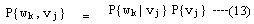Thus, either the joint or conditional probabilities may be used in the decision rule: R3 : Choose k which minimizes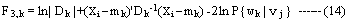Results
In the Moghan, crop production activities almost have disciplines, i.e. most farmers practice crop rotation to increase their yields, the rotational patterns, combined with information about crops-planted in the previous years, can be used to predict the current crop. The five years crop rotation matrix (transition matrix) is presented in Table 3.

Table 3: Transition Matrix in 5 consequent years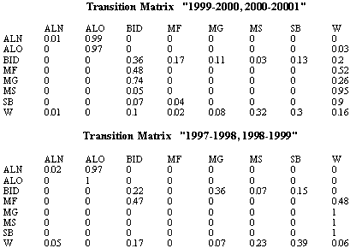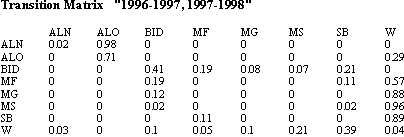Then by using produced transition matrices, which were described above and “1998-1999, 1999-2000” transition matrix (for estimating a prior probability) the classification calculated once again and validation results show that overall accuracy goes to 63%.

Conclusions
This paper has pointed out limitations of classification by MLM, and then proposed a classification method by considering a prior probability with discovering crop rotation schemes. Validation showed that the proposed method gave higher classification accuracy than traditional MLM. Further research must be conducted to explore other external knowledge to be included in the procedure and enhance the results.

References

• Abkar, A. 1999. Likelihhod-based segmentation and classification of remotely sensed Images. Ph.D. Thesis, University of Twente, ITC, Enschede, The Netherlands.
• Alesheikh, Ali A. 1998. Handling Uncertainty in Object-Based GISs. Ph.D. Thesis, Departmene of Geomatics Engineering, The University of Calgary, AB, Canada
• Gorte, B. 1998. Probability segmentation of remotely sensed images. Ph.D. thesis. Wayeningen Agricultural University (WAU), ITC, Enschede, The Netherlands.
• Richards, J.A. 1993. Remote Sensing Digital Image Analysis: An Introduction. Second Edition, Springer, ISBN 0-387-5480-8